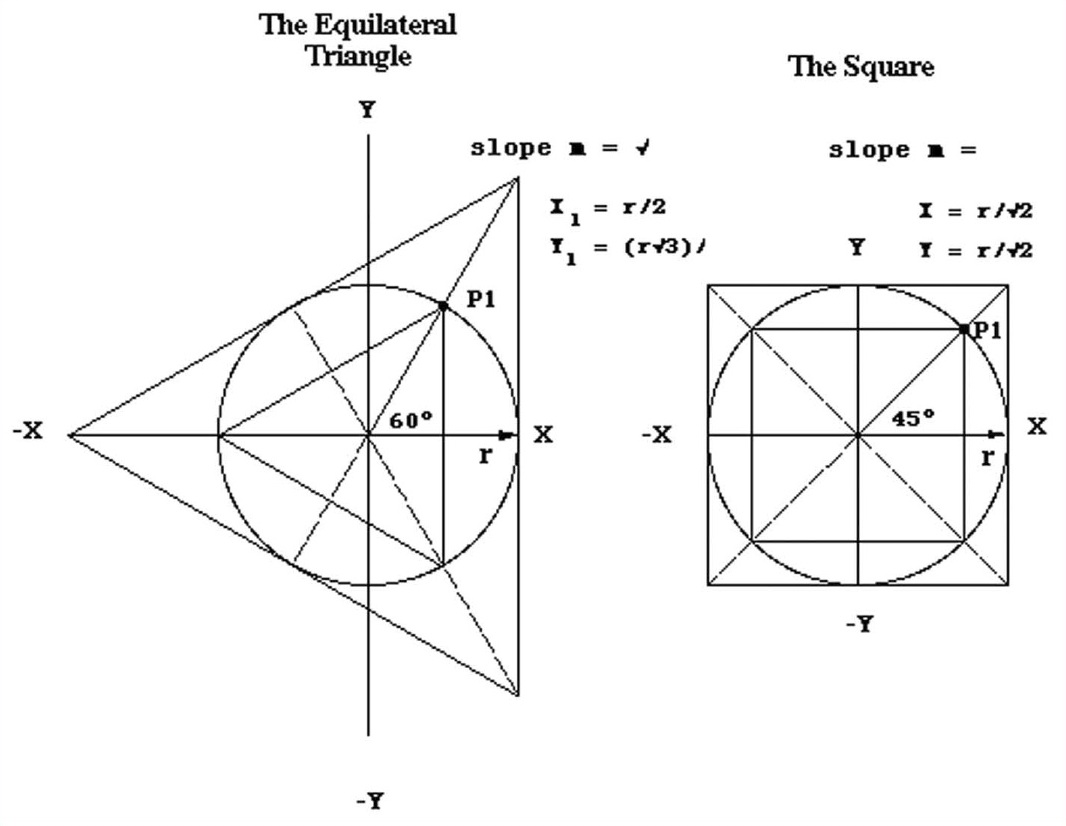also Squaring of the Circle.

"The vapor from the liberator, registered at 20,000 lbs. per square inch has a range of atomic motion of 1333 1/3 the diameter of the atmospheric molecule with constant rotary vibratory action. At 10,000 lbs., 666 2/3, at 5,000, 333 1/3, at 2500, 166 2/3, at 1250, 83 1/3, at 625, 41 2/3. The higher the range of atomic motion the greater its tenuity and pressure. The very evolution on the negative shows a vacuum of a much higher order than was ever produced before confounding all theory to analyze. The highest vacuum known is 17.999999, or not quite 30 inches, but Keely produced etheric vacuums repeatedly of 50 to 57 inches ranging down to 30 inches or 57 lbs. All operations of nature have for their sensitizing centers of introductory action, triple vacuum evolutions. These evolutions are centered in atomic triple revolutions, highly radiophonic in their character and thoroughly independent of all outside forces in their spheres of action. No conceivable power, however great, can break up their independent centers. These triple centers are the foundation of the universe, and mathematically considered, the respective and relative motion of these atomic triplets, gravitating to and revolving around each other, is about one and one-third of their circumference. The problem of this action, when analyzed mathematically, (taking it as the quadrature of the circle) would baffle mathematical science to bring it to a numerical equation. Every revolving body is impressed by nature with certain laws making it susceptible of the operation of force, which being applied, impels motion. These bodies never can approach nearer than a certain limit, nor farther than a certain point. They are, at some mean point, made perfectly equal, and may therefore be considered as one force and as one element. It matters not that other and disturbing forces exist outside or inside the space these bodies revolve in, because if this force must be considered as acting uniformly, applying itself to each of these bodies in a way to produce a perfect equation on all, it is as if this outside force were nonexisting." [JOHN ERNST WORRELL KEELY]

Treatise on the geometry and mathematics of Squaring of the Circle: Quadrature of the Circle

"In QUADRATURE OF THE CIRCLE Revealing THE TRUE VALUE OF Pi, we explore and expand upon Mr. Parker's findings. In clear, up to date English the central principles of the circle are revealed which allow the true and correct value of Pi to be calculated. Several important characteristics of the the arc, straight line, circle, triangle and regular polygon which have not been given due recognition are explored. Methods for constructing each geometric shape are given and the great importance of the Pythagorean or 'right' Triangle described. General formulas are developed allowing any regular shape to be made equal to another by area, perimeter, or proportion." Quadrature of the Circle George Hull's book

Cubing the Sphere - 3D version of squaring the circle